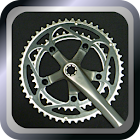Gear Ratio Calculator

All Android applications categories

All Android games categories# Gear Ratio Calculator

by: 2 9

9 Users
rating

## Screenshots

Description

This application calculates gear ratios for the road bike and the MTB.
Combination of 22 types of chain wheel, 39 types of sprockets and 70 types of tires - Create a graph of the gear ratio and speed-cadence.
By switch ON/OFF the display of each inner / center / outer gear, you can simulate the optimal gear ratio.
This application helps you to choosing a satisfy replacement parts.

--------------
This application allow the simulation of the gear ratio in the four charts.

[km/h RPM]
[mph RPM]
[m/s RPM]
Create a praph, the speed (km/h, mph, m/s) values will be displayed on the vertical axis, and the cadence (rpm) values will be displayed on the horizontal axis.
This graph can be displayed the changes of the speed for each position of the gear in revolutions of the crank.
The gear ratio to match the speed range you want, while viewing a graph, you can simulate a combination of parts.

[Gear Ratio]
Calculates and displays the gear ratio from each position of the selected chain wheel and sprocket.
Increases the burden on the foot when shifting between long distance between points on the graph, the smaller it is close.

[Gain Ratio]
To caliculate and display the distance run by the crank comes full circle.

-------------------
* We'll add the data of chain wheel, sprocket and tire.

Tags: application of gear ratio , bike gear ratio calculator , gear positions , gear ratio crank gear , gear rat bug calculate , how to calculate meters per second with gear ratio , rpm to m/s calculator gear

from 2 reviews

"Awesome"

9